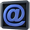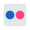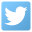###### Daily, archived, Desktop Wallpaper today=new Date(); // Initialize Date in raw form date=today.getDate(); // Get the numerical date year=today.getFullYear(); // Get the year day = today.getDay(); // Get the day in number form (0,1,2,3,etc.) month=today.getMonth()+1; // Get the month // Make day number value correspond to actual day name var dayName=new Array(7) dayName="Sunday"; dayName="Monday"; dayName="Tuesday"; dayName="Wednesday"; dayName="Thursday"; dayName="Friday"; dayName="Saturday"; // Add suffix to date (1st, 2nd, 4th, etc.) if (date==1) suffix=("st"); else if (date==2) suffix=("nd"); else if (date==3) suffix=("rd"); else if (date==21) suffix=("st"); else if (date==22) suffix=("nd"); else if (date==23) suffix=("rd"); else if (date==31) suffix=("st"); else suffix=("th"); // Make month number correspond to month name if (month==1) monthName=("January"); else if (month==2) monthName=("February"); else if (month==3) monthName=("March"); else if (month==4) monthName=("April"); else if (month==5) monthName=("May"); else if (month==6) monthName=("June"); else if (month==7) monthName=("July"); else if (month==8) monthName=("August"); else if (month==9) monthName=("September"); else if (month==10) monthName=("October"); else if (month==11) monthName=("November"); else monthName=("December"); // Write date document.write(dayName[day] + ", " + date + suffix + " " + monthName + " " + year);

Click on any image on this page to load the full sized wide-screen picture and save as you would for any other wallpaper pictures on this website.

###### (Wallpaper help page)

Note: There are now more 180 desktop wallpapers on these web page, plus seasonal desktop wallpaper each day as 'Picture of the Day' from previous holidays. I hope that you enjoy viewing and using these. If you are a fan of the downloadable Windows jigsaws, please check here!

###### More wallpaper pages. See the page links below, bottom left

•E-mail
•Flickr
•Twitter